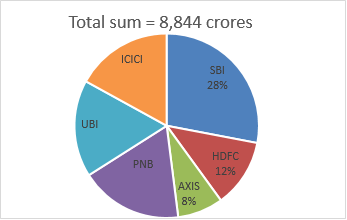+ -

## GK Trick

### Quantitative Aptitude Quiz For NIACL AO Prelims: 2018

Directions (1-5): The following pie-chart shows the percentage distribution of sum of six different banks with which an Indian businessman, Vijay Malya, fled away from India. Study the chart carefully to answer the following questions.

Note : In pie-chart some data are missing. If required, find them first and then proceed.Q1. If the sum from PNB was 250/3% more than the sum from HDFC bank, then find the sum from PNB with which Malya fled away from India ?
1968.45 crores
1945.68 crores
1519.68 crores
1845.84 crores
None of these

Q2. If the average sum from ICICI, SBI and Axis banks together with which Malya fled away from India, was Rs. 1,474 crores, then find the sum from ICICI bank that Malya took away from country ?
1084.26 crores
1343.16 crores
1238.16 crores
1440.24 crores
None of these

Q3. What was the total sum from HDFC, PNB and UBI together with which Malya fled away from country(for ICICI bank use the value obtained in Q2.) ?
3,944 crores
4,544 crores
3,842 crores
4,422 crores
None of these

Q4. Find the ratio of money from SBI and UBI together to the money from PNB and ICICI banks together if percentage value of PNB is 6% more than that of UBI.(use ICICI bank data from previous question)
4 : 9
9 : 11
11 : 9
9 : 4
None of these

Q5. If the percentage distribution of money of SBI and PNB was interchanged, then find the average sum from PNB, UBI and ICICI together with which Malya fled away from India.(If required,Use the value of missing data found in above questions)
1810.84 crores
1709.84 crores
1725 crores
1764.64 crores
None of these

Q6. The simple interest accrued on an sum of Rs 27,500 at the end of three years is Rs 10,230. What would be the approximate compound interest accrued on the same sum at the same rate in two years?
Rs 7,550
Rs 7,243
Rs 8,950
Rs 6,900
Rs 7,583

Q7. Out of 5 women and 4 men a committee of four members is to be formed in such a way that at most three members are men. In how many different ways can it be done?
125
120
105
130
None of these

Q8. A is thrice as good a workman as B and therefore is able to finish a job in 30 days less than B. How many days will they take to finish the job working together?
10¼
11¾
11¼
11⅓
None of these

Q9. A certain product C is made of two ingredients A and B in the proportion of 2 : 5. The price of A is three times that of B. The overall cost of C is Rs. 5.20 per kg including labour charges of 80 paisa per kg. Find the cost of B per kg ?
Rs. 8.40
Rs. 4.20
Rs. 4.80
Rs. 2.80
None of these

Q10. Parameshwaran invested an amount of Rs 12000 at the simple interest rate of 10 per cent annum and another amount at the simple interest rate of 20 per cent per annum. The total interest earned at the end of one year on the total amount invested became 14 per cent per annum. Find the total amount invested.
Rs 22000
Rs 25000
Rs 20000
Rs 24000
None of these

Directions (11-15): Find the missing term in the following number series.

Q11. 100, 49, 99, 48, 98, ?
51
50
49
48
47

Q12. 0, 7, 26, 63, 124, ?
219
215
205
225
227

Q13. 10, 21, 43, 87, 175, ?
349
350
351
352
347

Q14. 2, 3, 5, 8, 12, 17, ?
22
23
24
25
26

Q15. 1, 11, 29, 56, 93, 141, ?
200
201
202
203
205

SOLUTIONS

1.B
2.C
3.D
4.C
5.B
6.B
7.A
8.C
9.D
10.C
11.E
12.B
13.C
14.B
15.B

5 Banking and SSC : Quantitative Aptitude Quiz For NIACL AO Prelims: 2018 Directions (1-5): The following pie-chart shows the percentage distribution of sum of six different banks with which an Indian businessman,...